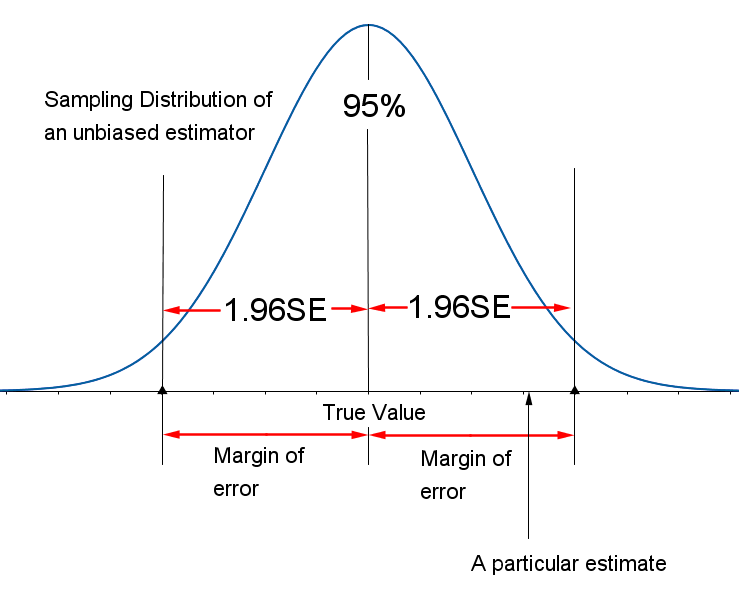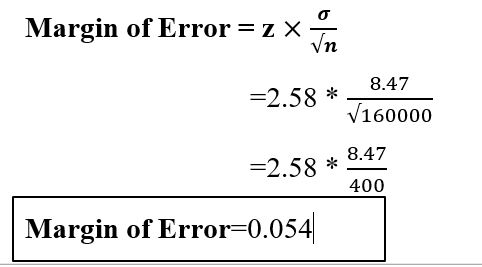### Margin Of Error

• date 11th July, 2019 |
• by Prwatech |

# Margin Of Error tutorial

Margin of error tutorial, hunting for the best platform which provides information about a margin of error statistics? Then welcome to the land of a margin of error tutorial on Data Science. In this tutorial one can explore a margin of error definition in data science with examples and how is the margin of error calculated which were prepared by India’s Leading Data Science training institute professionals.

If you are the one who wanted to become an expert in Data Science? Or the one who wanted to explore the technology like a Pro under the certified experts with a world-class classroom training environment, then starts taking Data Science training from Prwatech who can help you to guide and offer excellent training with highly skilled expert trainers. Follow the below-mentioned margin of error tutorial and enhance your skills to become a professional Data Scientist.

## what is the margin of error in statistics?

The margin of error is a range of values below and above the sample statistic in a confidence interval.

The margin of error is an interval estimate is a pair of percentages surrounding a guess about some attribute of full population-based over a random sample from that population.

“Margin of error allows us to feel confident a certain percentage of the time, within a range above or below the ideal guess, represented by a margin we believe is least in error”In probability sampling, every member of a population has a probability of being selected to be a part of a sample. In this method, researchers and statisticians can select members from their area of research so that a margin of error in data received from these samples is as minimum as possible.

In non-probability sampling, samples are formed over the basis of cost-effectiveness or convenience and not over the basis of application and because of this selection process, some sections of the population may get excluded.

Surveys will be effective only on filtering members according to interests and application to the survey being conducted.

### Why use the Margin of Error?

Whenever we use a representative sample to make a guess something about a full population, our guess would contain some kind of uncertainty.  Using our sample statistic, we have to conclude the real statistic—and that inference will mean our guess will usually be somewhere near the actual figure (a bit too low or a bit too high, Statistics Solutions).

### How is the margin of error calculated?

Margin of Error = z

Where n= sample size

σ= Population Standard Deviation

z= z-score

### Margin of error Formula with Examples

Find Margin of Error where sample size = 160000, Population Standard Deviation= 8.47 and z-score= 2.58Grab knowledge of the concepts of a margin of error statistics. Prwatech is the best-trusted E-learning for Data science training in Bangalore. Our expert trainers will help the aspirants in grabbing clear cut knowledge of all the advanced applications of Data Science.

### Quick Support